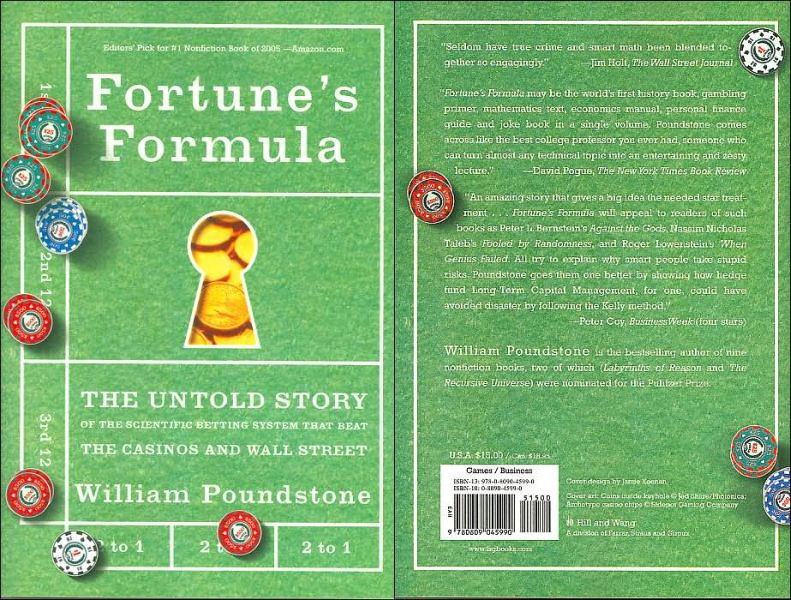# Calculate Fortune's Formula with PerlThe Kelly criterion is an equation for deriving the optimal fraction of a bankroll to place on a bet, given the probability and the betting odds. I read about it a few years ago in William Poundstone’s page turner, Fortune’s Formula. To use the Kelly criterion in Perl code, you can used Algorithm::Kelly, a module I released last week.

### Using Algorithm::Kelly

Algorithm::Kelly exports the `optimal_f` sub, which takes two parameters: the probability of the event occurring (a value between 0.00 and 1.00) and the payoff (net betting odds). `optimal_f` returns the optimal fraction of your betting bankroll to place on the bet.

For example if I want to find the optimal f of a bet which has a 50% chance of winning, and pays 3-to-1:

``````use Algorithm::Kelly;

my \$optimal_f = optimal_f(0.5, 3);``````

Here `optimal_f` returns a value of `0.25`, which means I should place 25% of my bankroll on this bet. Let’s look at another example: a bet which has 12% chance of occurring and pays 5-to-1. I can also calculate optimal f at the command line:

``````\$ perl -MAlgorithm::Kelly -E 'say optimal_f(0.12, 5)';
-0.056``````

So this time, optimal f is `-0.056`, or negative 5.6%, which means I shouldn’t take this bet as the odds are not generous enough, given the probability of the bet winning. This is tremendously useful: the optimal fraction can be used to eliminate bad bets, and also rank competing betting options, to find the best value bet.

### Practical pitfalls

The Kelly criterion is only as accurate as its inputs, and whilst it’s easy to look up the odds offered for a particular bet, precisely calculating the probability of the bet winning is usually a far more difficult task. It’s easy to calculate the probability for casino games like roulette, but they have negative optimal fs and are not worth pursuing. Some successful sport bettors use statistical modeling techniques to estimate the probability of a bet winning - but this is only an estimate.

The second issue with the Kelly criterion is the size of optimal f. The Kelly criterion will always maximize return over the long term, but there is not an infinite market of bets available, and regularly risking high percentages of your bankroll will mean a big short term losses. Further, even if you have a sizable bankroll, many markets are simply not liquid enough to accommodate the size of bets recommended by optimal f. Bettors will often use a “half-Kelly” instead, which is the optimal f of a bet divided by 2.

This article was originally posted on PerlTricks.com.

Tags

### David Farrell

David is the editor of Perl.com. An organizer of the New York Perl Meetup, he works for ZipRecruiter as a software developer, and sometimes tweets about Perl and Open Source.

### Feedback

Something wrong with this article? Help us out by opening an issue or pull request on GitHub